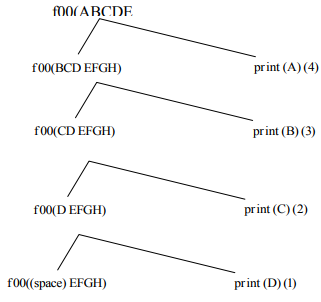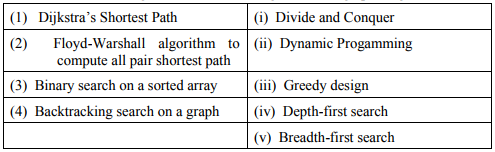# CS GATE 2015 - Online Test

Q1. Choose the statement where underlined word is used correctly.
Explaination / Solution:

personnel- people employed in an organization or engaged in an organized undertaking such as military service.

Q2. Based on the given statements, select the most appropriate option to solve the given question What will be the total weight of 10 poles each of same weight? Statements (I) One fourth of the weight of a pole is 15kg. (II) The total weight of these poles is 160 kg more than the total weight of two poles
Explaination / Solution:
No Explaination.

Q3. Out of the following four sentences, select the most suitable sentence with respect to grammar and usage.
Explaination / Solution:

(B) there was no needed information (C) not really useful (D) would not have been

Q4. An unordered list contain n distinct elements. The number of comparisons to find an element in this list that is neither maximum nor minimum is
Explaination / Solution:

Consider first three element of the list, atleast one of them will be neither minimum nor maximum ϴ(1)

Q5. Consider the following function written the C programming language.
void foo (char *a) {
if (*a && *a ! = ' ') {
putchar (*a) ;
}
}
The output of the above function on input “ABCD EFGH” is
Explaination / Solution:if condition fails & returns controls
DCBA will be pointed

Q6. Given below are some algorithms, and some algorithm design paradigmsMatch the above algorithms on the left to the corresponding design paradigm they follow.
Explaination / Solution:

Dijkstra shortest path algorithm find next node by choosing minimum distance hence greedy approach. Floyd warshall always apply dynamic programming, once it saves a cost and in the next iteration it will change if this is minimum hence dynamic. Binary search always divide on two parts .Hence divide and conquer. Backtracking uses by DFS, one it will reach to dead end it will take back track.

Q7.  Suppose you are provided with the following function declaration in the C programming language
int partition (int a [ ], int n);
The function treats the first element of a [ ] as a pivot, and rearranges the array so that all elements less than or equal to the pivot is in the left part of the array , and all elements greater than the pivot is in the right part. In addition, it moves the pivot so that the pivot is the last elements of the left part. The return value is the number of elements in the left part.
The following partially given function in the C programming language is used to find the Kth smallest element in an array a [ ] of size n using the partition function We assume k ≤ n.
int kth _smallest (int a [ ], int n, int k)
{
int left _ end = partition (a,n);
if (left _ end + 1 == k) {
return a [left _ end];
}
if (left _ end + 1 > k) {
return kth _ smallest (__________________);
}else {
return kth_smallest (_______________________);
}
}
The missing argument lists are respectively
Explaination / Solution:
No Explaination.

Q8. Consider a simple checkpointing protocol and the following set of operations in the log.
(Start, T4); (write, T4,y,2,3); (Start, T1); (commit,T4); (write, T1,z,5,7);
(checkpoint);
(Start,T2); (write, T2,x,1,9); (commit,T2); (start,T3), (write,T3,z,7,2);
If a crash happens now and the system tries to recover using both undo and redo operations, what are the contents of the undo lists and the redo list?
Explaination / Solution:

As T1 & T3are not yet committed they must be undone. The transactions which are after the latest checkpoint must be redone. So T2 must be redone. No need to redo the records which are before last checkpoint, so T4 need not be redone.

Q9. The secant method is used to find the root of an equation f(x) = 0. It is started from two distinct estimates xa and xb for the root. It is an iterative procedure involving linear interpolation to a root. The iteration stops if f(xb) is very small and then xb is the solution. The procedure is given below. Observe that there is an expression which is missing and is marked by? Which is the suitable expression that is to be put in place of? So that it follows all steps of the secant method?
Initialize: xa, xb, ε, N                                 // ε = convergence indicator
fb = f(xb)
i = 0
while (i < N and | fb | > ε) do
i = i + 1                                                     // update counter
xt = ?                                                        // missing expression for // intermediate value
xa= xb                                                      // reset xa
xb = xt                                                      // reset xb
fb = f(xb)                                                  // function value at new xb
end while
if |fb| > ε then                                          // loop is terminated with i = N
write “Non-convergence”
else
write “return xb”
end if

Explaination / Solution:

Secant method direct formula

Q10. Which one of the following hash functions on integers will distribute keys most uniformly over 10 buckets numbered 0 to 9 for i ranging from 0 to 2020?
Explaination / Solution:

If we take first 10 elements, number of collisions taken by the hash function given by option (B) is less when compared to others.